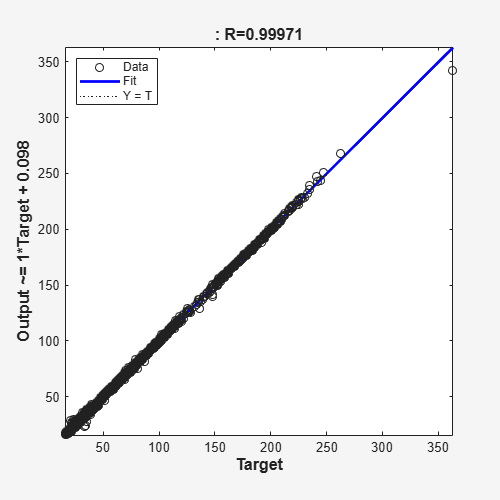# network

Class: Autoencoder

Convert `Autoencoder` object into `network` object

## Syntax

``net = network(autoenc)``

## Description

example

````net = network(autoenc)` returns a network object which is equivalent to the autoencoder, `autoenc`.```

## Input Arguments

expand all

Trained autoencoder, returned as an object of the `Autoencoder` class.

## Output Arguments

expand all

Neural network, that is equivalent to the autoencoder `autoenc`, returned as an object of the `network` class.

## Examples

expand all

`X = bodyfat_dataset;`

X is a 13-by-252 matrix defining thirteen attributes of 252 different observations. For more information on the data, type `help bodyfat_dataset` in the command line.

Train an autoencoder on the attribute data.

`autoenc = trainAutoencoder(X);`Create a network object from the autoencoder, `autoenc` .

`net = network(autoenc);`

Predict the attributes using the network, `net` .

`Xpred = net(X);`

Fit a linear regression model between the actual and estimated attributes data. Compute the estimated Pearson correlation coefficient, the slope and the intercept (bias) of the regression model, using all attribute data as one data set.

`[C, S, B] = regression(X, Xpred, 'one')`
```C = 0.9997 ```
```S = 0.9984 ```
```B = 0.0960 ```

The correlation coefficient is almost 1, which indicates that the attributes data and the estimations from the neural network are highly close to each other.

Plot the actual data and the fitted line.

`plotregression(X, Xpred);`The data appears to be on the fitted line, which visually supports the conclusion that the predictions are very close to the actual data.

## Version History

Introduced in R2015b